Question

# problem #4 #7 4. Problem A long wire is bent to form a rectangular section and is placed in a region with a uniform...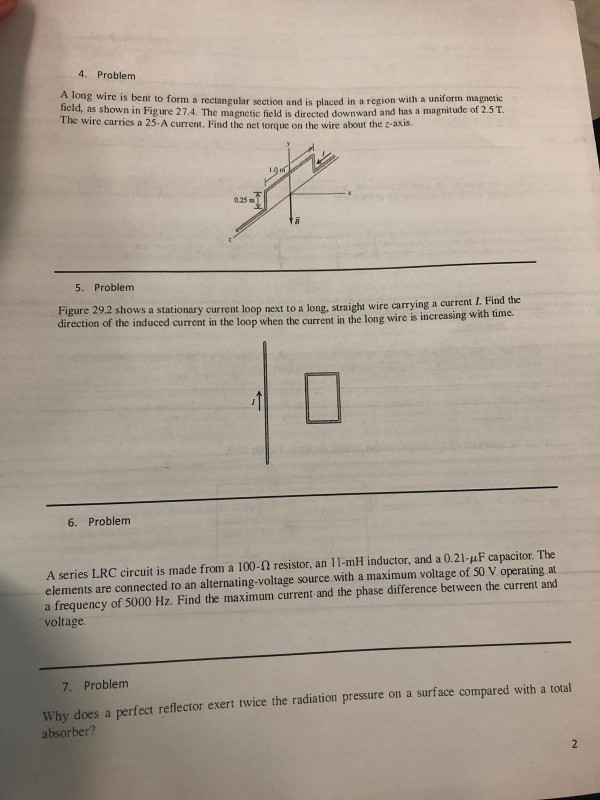problem #4 #7

4. Problem A long wire is bent to form a rectangular section and is placed in a region with a uniform magnetic id, as shown in Fig ure 27.4. The magnetic field is directed downward and has a magnitude of 2.5T. The wire carries a 25- A current. Find the net torque on the wire about the z-axis. 025m 5. Problem Figure 29.2 shows a stationary current loop next to a long, straight wire carrying a current direction of the induced current in the loop when the current in the long wire is increasing 6. Problem A series LRC circuit is made from a 100-Ω resistor, an 11-mH inductor, and a 0.2 ljuF capacitor. The elements are connected to an alternating-voltage source with a maximum voltage of 50 V operating at a frequency of 5000 Hz. Find the maximum current and the phase difference between the current and voltage 7. Problenm Why does a perfect reflector exert twice the radiation pressure on a surface compared with a total absorber?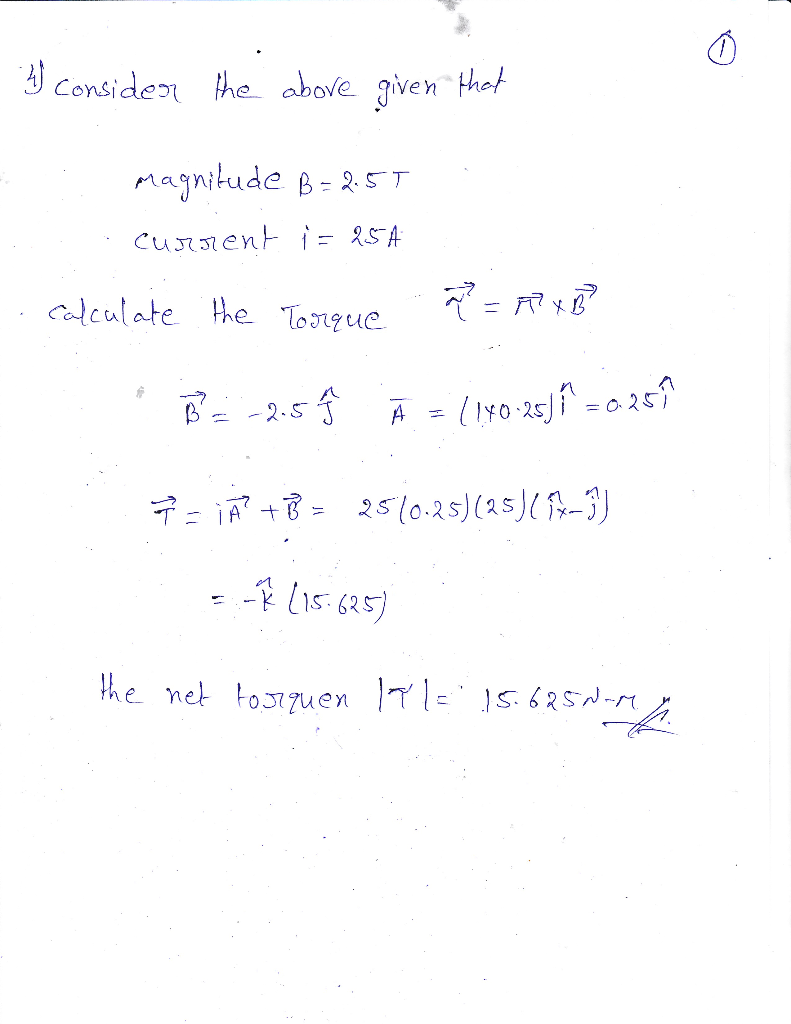#### Earn Coins

Coins can be redeemed for fabulous gifts.

Similar Homework Help Questions
• ### 4 Problem long wire is bent to form a rectangular section and is placed in sregion with a uniform naietis field, as shown in Figure 27.4. The magnetic field is directed downward and has a ma...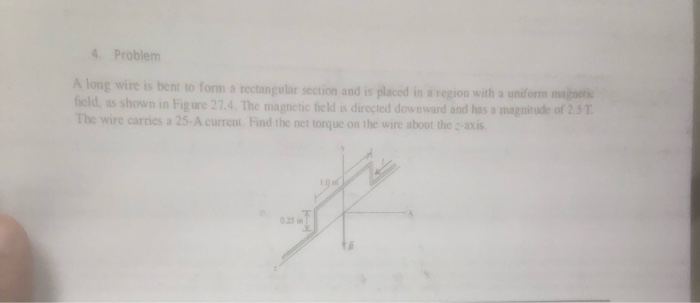4 Problem long wire is bent to form a rectangular section and is placed in sregion with a uniform naietis field, as shown in Figure 27.4. The magnetic field is directed downward and has a magnitude of 2.5 τ The wire carries a 25-A curent Find the net torque on the wire about the axis. 025 m to form shown carries a 25-A 025 m 4 Problem long wire is bent to form a rectangular section and is placed in...

• ### The bent wire shown in the figure lies in a uniform magnetic field. Each straight section is 2.78 m long and makes an angle of θ=42.1° with the x axis, and the wire carries a current of 1.21 A.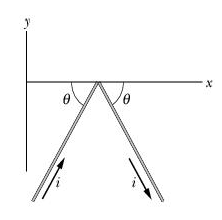Chapter 28, Problem 042 The bent wire shown in the figure lies in a uniform magnetic field. Each straight section is 2.78 m long and makes an angle of θ=42.1° with the x axis, and the wire carries a current of 1.21 A. What is the net magnetic force on the wire in unit-vector notation if the magnetic field is given by (a)3.91 kT? (b)3.75 İ T?

• ### Problem 4 Magnetic field region Fig. 2 A long wire carrying 4.50 A of current makes...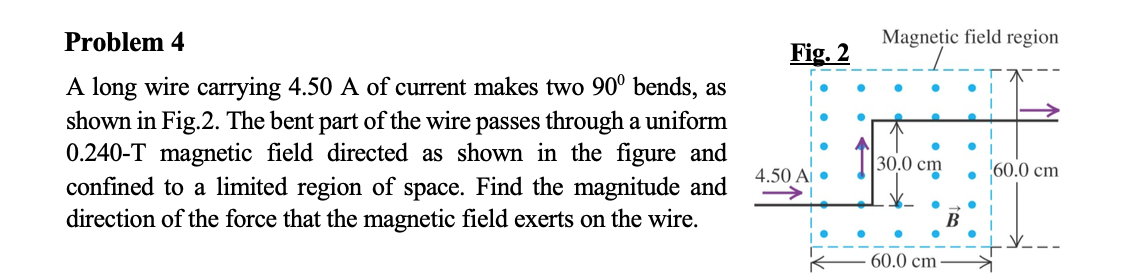Problem 4 Magnetic field region Fig. 2 A long wire carrying 4.50 A of current makes two 90° bends, as shown in Fig.2. The bent part of the wire passes through a uniform 0.240-T magnetic field directed as shown in the figure and confined to a limited region of space. Find the magnitude and direction of the force that the magnetic field exerts on the wire. . ||30.0 cm 60.0 cm 4.5 Y_ - 60.0 cm

• ### Problem 5: A 5 cm long wire is in a uniform magnetic field of 4T, so...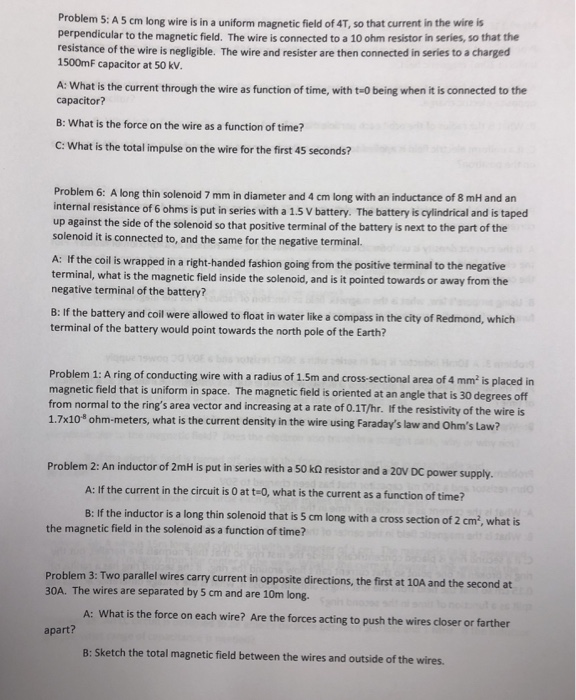Problem 5: A 5 cm long wire is in a uniform magnetic field of 4T, so that current in the wire is perpendicular to the magnetic field. The wire is connected to a 10 ohm resistor in series, so that the resistance of the wire is negligible. The wire and resister are then connected in series to a charged 1500mF capacitor at 50 kV. A: What is the current through the wire as function of time, with t-0 being when...

• ### 1) Figure 1 shows an infinity long wire with current I in +x direction. A triangular...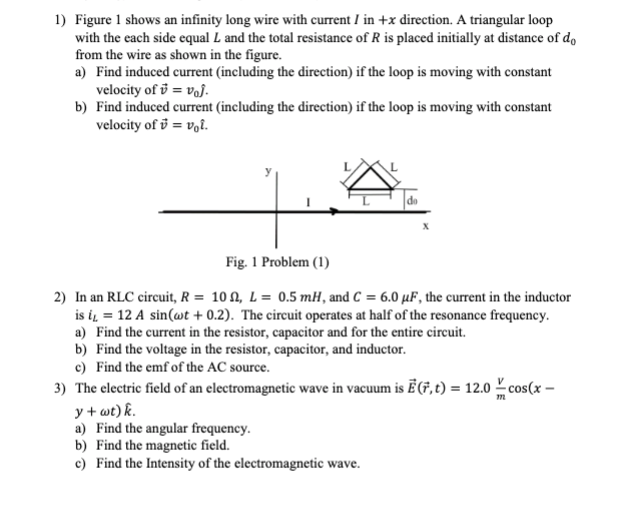1) Figure 1 shows an infinity long wire with current I in +x direction. A triangular loop with the each side equal L and the total resistance of R is placed initially at distance of do from the wire as shown in the figure. a) Find induced current (including the direction) if the loop is moving with constant velocity of v = voj. b) Find induced current (including the direction) if the loop is moving with constant velocity of j...

• ###  2. In the previous problem, how long after switch is closed until the magnetic field...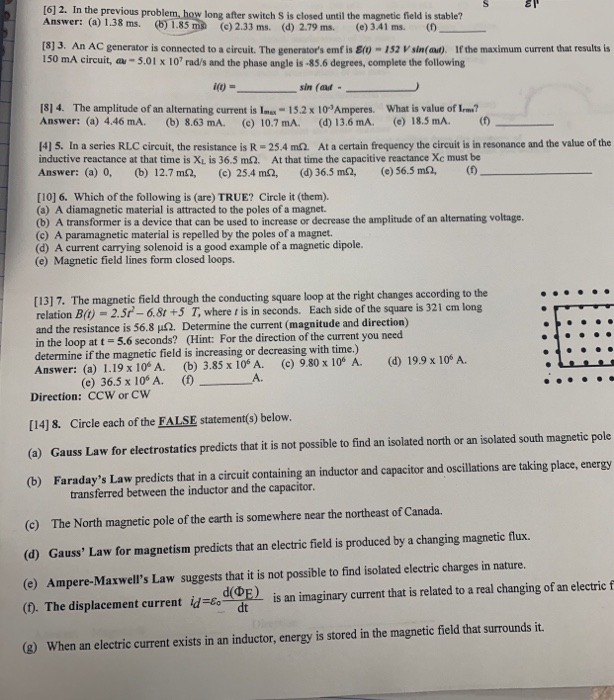2. In the previous problem, how long after switch is closed until the magnetic field is stable? Answer: (a) 1.38 ms. (6) 1.85 ms (c) 2.33 ms. (d) 2.79 ms. (e) 341 ms. (1) -  3. An AC generator is connected to a circuit. The generator's emfis E(0) - 152 V sin(at). If the maximum current that results is 150 mA circuit, -5.01 x 10rad/s and the phase angle is -85.6 degrees, complete the following (0) = sin...